### Home > ACC6 > Chapter 7 Unit 7 > Lesson CC1: 7.3.4 > Problem7-123

7-123.

ELEVATOR CHALLENGE

Juan got on an elevator at the middle floor of a building, went up $4$ floors, down $3$ floors, up $1$ floor, and down $9$ floors, where he left the elevator on the ground floor.

1. How many floors are in the building?

First, draw a vertical number line and pick a tick mark to represent the middle floor. A vertical number line with the middle tick mark, labeled, middle floor.

Then trace his path, starting at the middle floor and move $4$ floors up. Movement of 4 up, from the middle floor.

Then move $3$ floors down. Movement of 3 down, from the last position.

Next, move $1$ floor up. Movement of 1 up, from the last position.

Last, move $9$ floors down to the ground floor. Movement of 9 down, from the last position.

If you stop on the first floor and there are seven floors below the middle floor, there should be $7$ floors above. How many floors are there? Numbers, 1 to 7, are labeled on each tick mark, starting with 1 from the last position.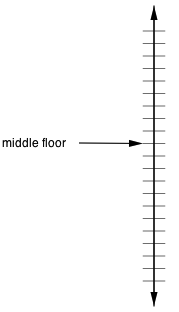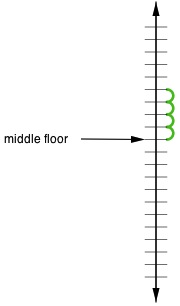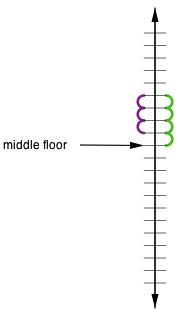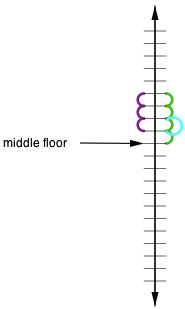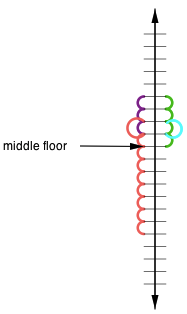2. Explain how you found the number of floors.

Did you work backwards?
Or did you set up a number line?